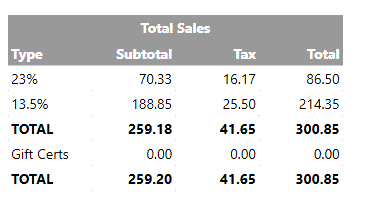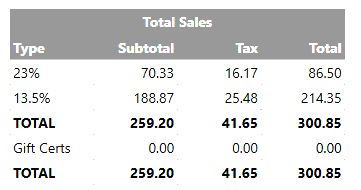# Report Ticket total breakdown by tax type

I am trying the fix a report that I need for tax returns with individual breakdown of sales under each tax type.

``````[Total Sales:1, 1, 1, 1]
>Type|Subtotal|Tax|Total
23%|{REPORT TICKET DETAILS:=[TXT.23% Vat.sum]/123*100,=[TXT.23% Vat.sum]/123*23,TXT.23% Vat.sum,:}
13.5%|{REPORT TICKET DETAILS:=[TXT.13.5% Vat.sum]/1135*1000,=[TXT.13.5% Vat.sum]/1135*135,TXT.13.5% Vat.sum,:}
>TOTAL|{REPORT TICKET DETAILS:=[TXT.23% Vat.sum]/123*100+[TXT.13.5% Vat.sum]/1135*1000,T.Tax.sum,=[TXT.23% Vat.sum]+[TXT.13.5% Vat.sum]:}
Gift Certs|{REPORT TICKET DETAILS:TA.GC Purchase Transaction.sum,TA.dummy.sum,TA.GC Purchase Transaction.sum:}
>TOTAL|{REPORT TICKET DETAILS:=[T.TotalAmount.sum]-[T.Tax.sum],T.Tax.sum,T.TotalAmount.sum:}
``````I originally used this report but there is always a slight discrepancy as i was using calculations to get the subtotal and tax totals. See actual subtotal at bottom 259.20 and calculated total is 259.18 a 2c discrepancy. Also if you add the Tax totals for both types there is also the 2c discrepancy 16.17+25.50 = 41.67 not the correct total of 41.65

Then as there was only 2 tax types I just used calculations for one `23% Vat` and then subtracted that from the total to get the 13.5%.

``````[Total Sales:1, 1, 1, 1]
>Type|Subtotal|Tax|Total
23%|{REPORT TICKET DETAILS:=[TXT.23% Vat.sum]/123*100,=[TXT.23% Vat.sum]/123*23,TXT.23% Vat.sum,:}
13.5%|{REPORT TICKET DETAILS:=[TXT.23% Vat.sum]+[TXT.13.5% Vat.sum]-[T.Tax.sum]-[TXT.23% Vat.sum]/123*100,=[T.Tax.sum]-[TXT.23% Vat.sum]/123*23,TXT.13.5% Vat.sum:}
>TOTAL|{REPORT TICKET DETAILS:=[TXT.23% Vat.sum]+[TXT.13.5% Vat.sum]-[T.Tax.sum],T.Tax.sum,=[TXT.23% Vat.sum]+[TXT.13.5% Vat.sum]:}
Gift Certs|{REPORT TICKET DETAILS:TA.GC Purchase Transaction.sum,TA.dummy.sum,TA.GC Purchase Transaction.sum:}
>TOTAL|{REPORT TICKET DETAILS:=[T.TotalAmount.sum]-[T.Tax.sum],T.Tax.sum,T.TotalAmount.sum:}
``````This is not correct but visually everything adds up even though technically the tax total wont always necessarily be exactly 23% or 13.5% of their respective totals.

The main problem I have now is I also need to add 9% VAT to this so I’m back to using calculations again.

I can get each tax type total using `TXT.23% Vat.sum`
Is it possible to add a new tag for just the actual tax total or even subtotal ex.tax?
Either of these can then be used to correctly calculate the other.

1 Like

Tax rounding when adding tax of individual transactions vs a % on total sales is inevitable.
The hotel has a variance between the two figures of between of arround 40-50p a day up to sometimes 1.30-1.40 on a busy friday/saturday.
The difference will increase with the number of transactions. As the hotel has a busy bar with hundreds of small one or two drink purchases the sub penny roundings soon add up and it is to be expected.

1 Like

Have you tried reporting order details rather than ticket details?
As tax is mapped to orders you may be able to get info needed that way. If not exc tax guess using total-tax…

Yes and thats what i am missing is this variance. I am just calculating exactly 23% and exactly 13.5% of total daily weekly sales etc. Which as you point out is never the case especially when doing bi monthly tax reports.

The example above is just from a couple of test sales so it only added up to a 2c discrepancy but you are misunderstanding what i am trying to point out. The bottom row is the correct total and tax total. It is not an exact percentage but varies.

The top rows are incorrect because i can only calculate exactly 23% or 13.5% from the info available. This is where there should be variance to match the bottom row.
If the information is there I dont see why it cant be pulled directly without doing “rough” calculations.
We have a tag for the t.tax.sum and then a tag for total sales for each tax type TXT.23% Vat.sum
All thats missing is the tax sum for each tax type.

Order details wont work as they don’t account for ticket discounts or cash rounding etc.

Did you tried `TX.23% Vat.sum` ?

2 Likes

Thanks I knew this data had to be available somewhere. I was looking on the wiki but didnt see anything.
Thanks for you help

1 Like